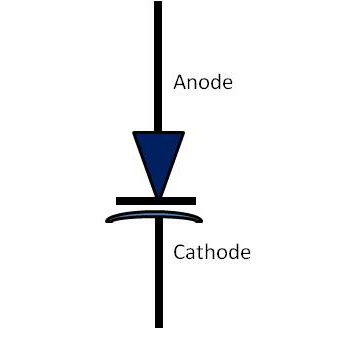# Semiconductor Devices - Varactor Diode

This is a special P-N junction diode with an inconsistent concentration of impurities in its P-N materials. In a normal PN junction diode, doping impurities are usually dispersed equally throughout the material. Varactor diode doped with a very small quantity of impurities near the junction and impurity concentration increases moving away from the junction.

In conventional junction diode, the depletion region is an area which separates the P and N material. The depletion region is developed in the beginning when the junction is initially formed. There are no current carriers in this region and thus the depletion region acts as a dielectric medium or insulator.

The P-type material with holes as majority carriers and N type material with electrons as majority carriers now act as charged plates. Thus the diode can be considered as a capacitor with N- and P-type opposite charged plates and the depletion region acts as dielectric. As we know, P and N materials, being semiconductors, are separated by a a depletion region insulator.

Diodes which are designed to respond to the capacitance effect under reverse bias are called varactors, varicap diodes, or voltage-variable capacitors.

The following figure shows the symbol of Varactor diode.Varactor diodes are normally operated in the reverse bias condition. When the reverse bias increases, the width of the depletion region also increases resulting in less capacitance. This means when reverse bias decreases, a corresponding increase in capacitance can be seen. Thus, diode capacitance varies inversely proportional to the bias voltage. Usually this is not linear. It is operated between zero and the reverse breakdown voltage.

The capacitance of Varactor diode is expressed as −

$$C_T = E\frac{A}{W_d}$$

• CT = Total capacitance of the junction

• E = Permittivity of the semiconductor material

• A = Cross-sectional area of the junction

• Wd = Width of the depletion layer

These diodes are variable used in microwave applications. Varactor diodes are also used in resonant circuits where some level of voltage tuning or frequency control is required. This diode is also employed in Automatic Frequency Control (AFC) in FM radio and television receivers.